# Basic Triangle Constructions

Basic Triangle Constructions
Go back to  'Triangles'

In this lesson we will learn about the definition of a triangle, how to construct triangles, how to construct equilateral triangles, altitude of a triangle construction, protractor, scale, triangle construction worksheet.

## Lesson Plan

 1 How to Construct Triangles? 2 Important Notes on Constructing Triangles 3 Thinking Out of the Box! 4 Solved Examples on Constructing Triangles 5 Interactive Questions on Constructing Triangles

## How To Construct Triangles?

A triangle is a two-dimensional closed shape with three sides, three edges and three vertices. A triangle can be constructed using a ruler, protractor and a compass. Constructing a triangle is very important while applying pythogoras theorem and in trigonometry. Triangles can be classified based on their sides and angles. We will discuss the steps, properties and criteria to construct various triangles.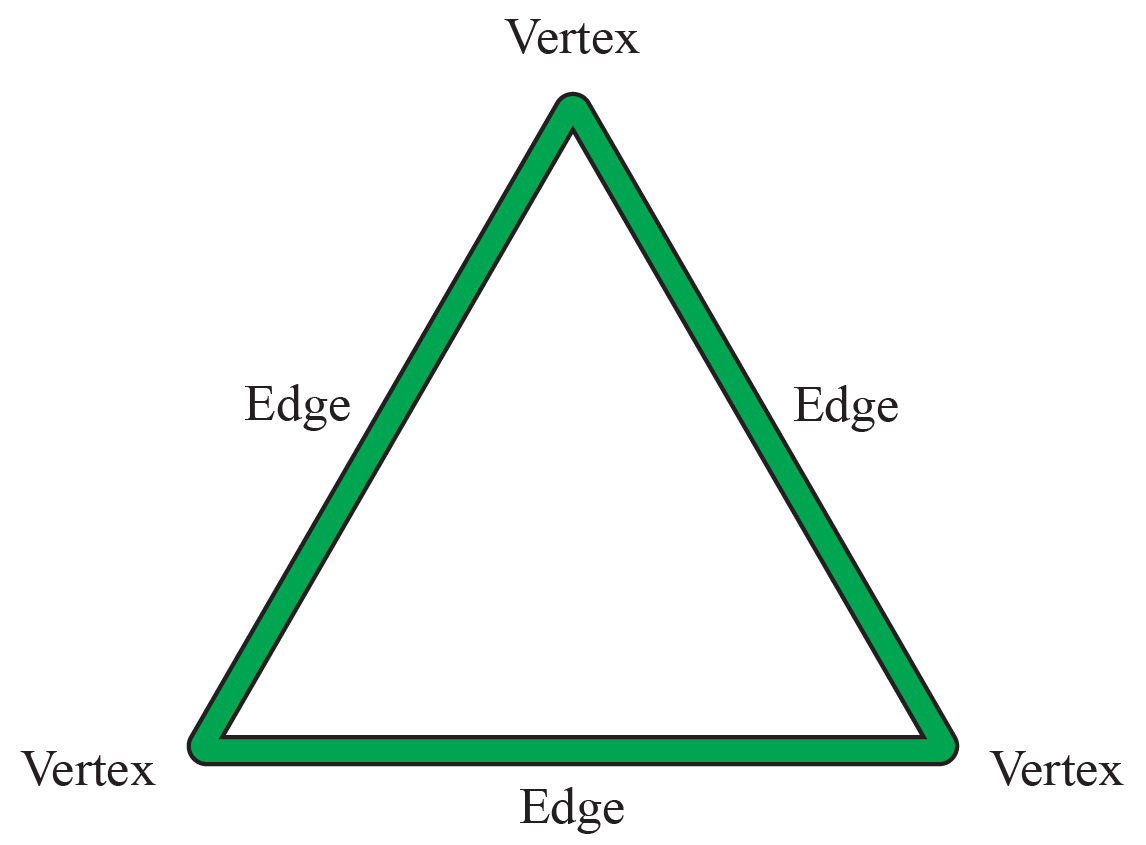Properties Of A Triangle

• A triangle has three sides,three vertices and three angles
• The sum of interior angles of a triangle is equal to 1800. This property is called the angle sum property of a triangle
• All the sides of a triangle may or may not be equal
• The sum of length of two sides of the triangle is always greater than the third side
• A triangle is with vertices A,B,C is denoted as triangle ABC
• The area of a triangle is equal to half of its base multiplied by height

## Types of Triangle

Triangles can be classified based on

• Angles
• Sides
• Angles and Sides

Triangles By Angles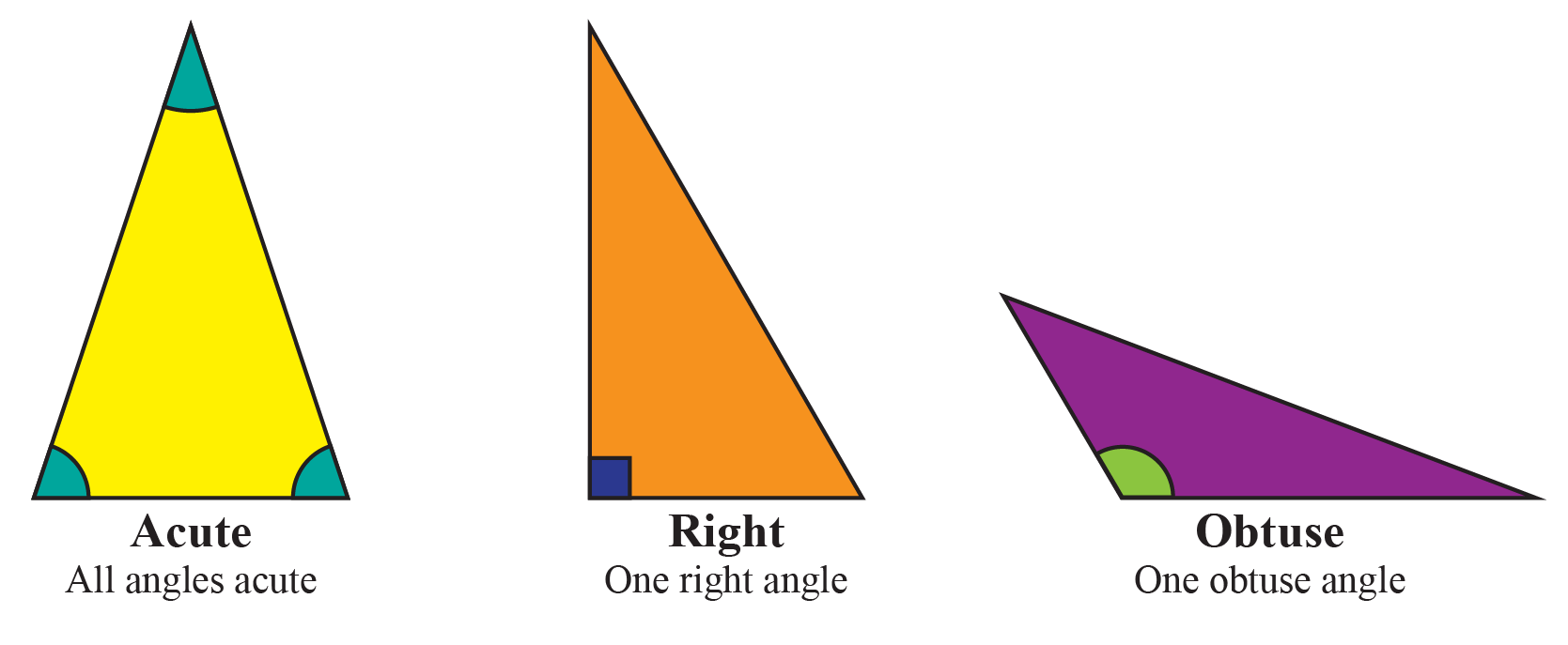An acute triangle has one acute angle (less than 900)
A right-angled triangle has one right angle (equal to 900)
An obtuse angle has one of its angles to be obtuse (greater than 900)

Triangle By Sides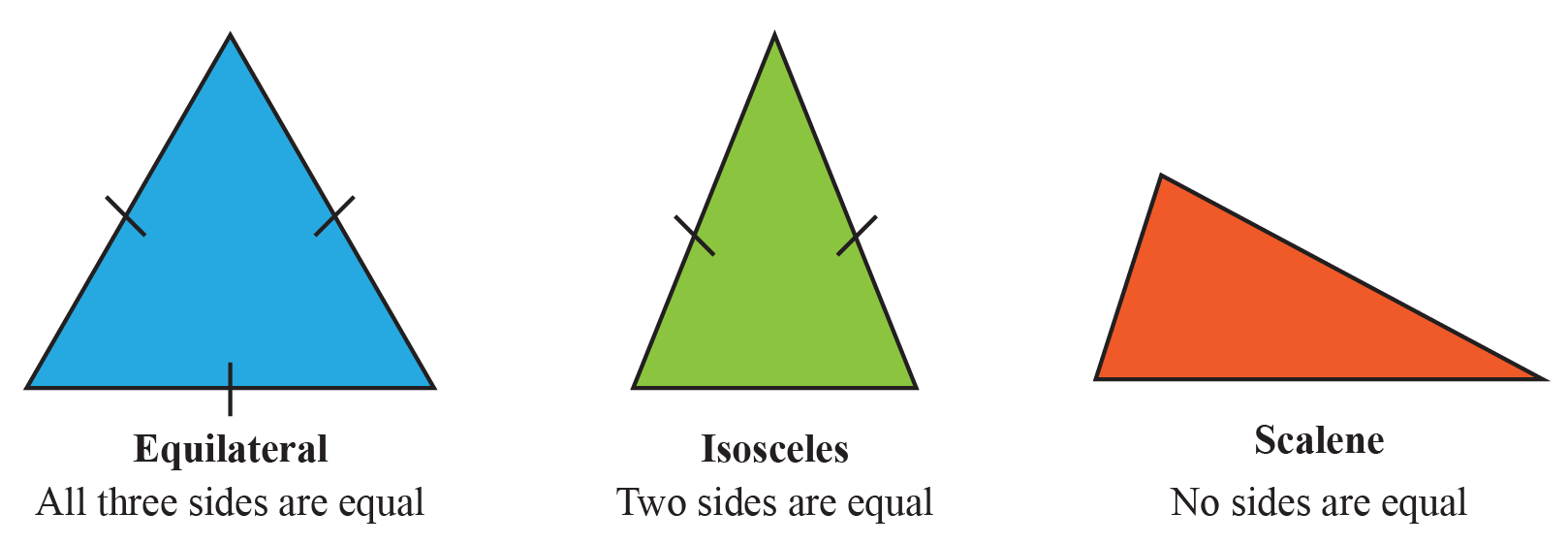An equilateral triangle has all of its sides to be equal.
An isosceles triangle has two sides equal.
A scalene triangle has three unequal sides.

Triangles By Different Sides And Angles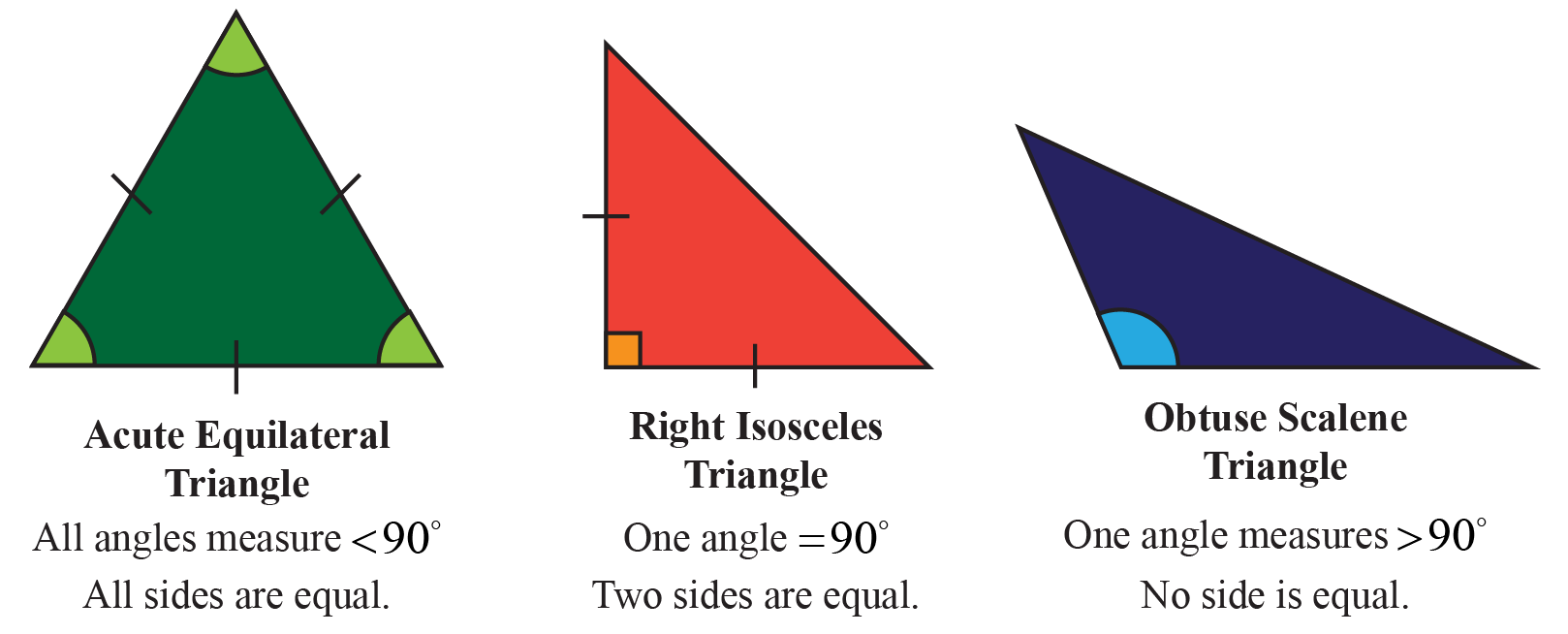An acute equilateral triangle has all angles lesser than 900. Also, all sides are equal.
A right isosceles triangle has one of its angles equal to 900. It has two sides to be equal.
An obtuse scalene triangle has one obtuse angle(greater than 900). All the sides are unequal.

## What Is The Meaning Of Triangle Construction?

As discussed triangle is a three-sided two-dimensional closed shape. In order to construct a triangle, we should know the measure of the length of its sides and angles.

But how do we construct them?

Here comes in the concept of 'Geometry'

Geometry is a branch of math that deals with constructing figures like triangles, squares, circles, and many other shapes. This can be done with geometrical instruments like a ruler, protractor (used to measure angles), and compasses.

Now let us jump into the steps of constructing a triangle.

## Steps To Construct A Triangle

Based on the properties of the triangle that we learned earlier in this lesson, we will start constructing them

Property 1: The sum of the length of any two sides of a triangle is greater than the third side.

Let us assume the sides of the triangle to be a,b and c.

Then \begin{align} (a+b) > c\: (or)\: (a+c) > b \:(or)\: (b + c) > a \end{align}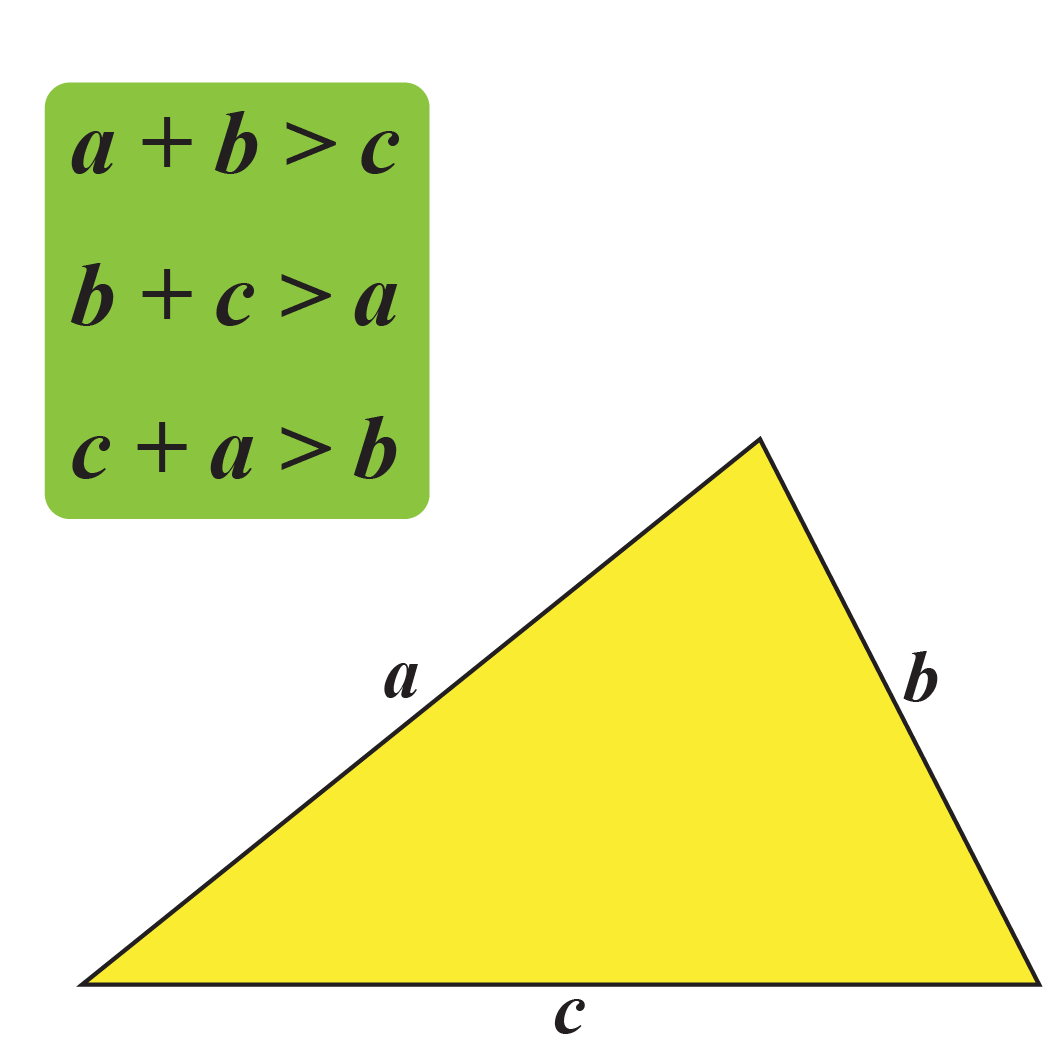Property 2: The sum of the interior angles of a triangle is equal to 1800

A triangle with three angles A, B and C has,

\begin{align}\angle{A} + \angle {B} + \angle {C} = 180^0\end{align}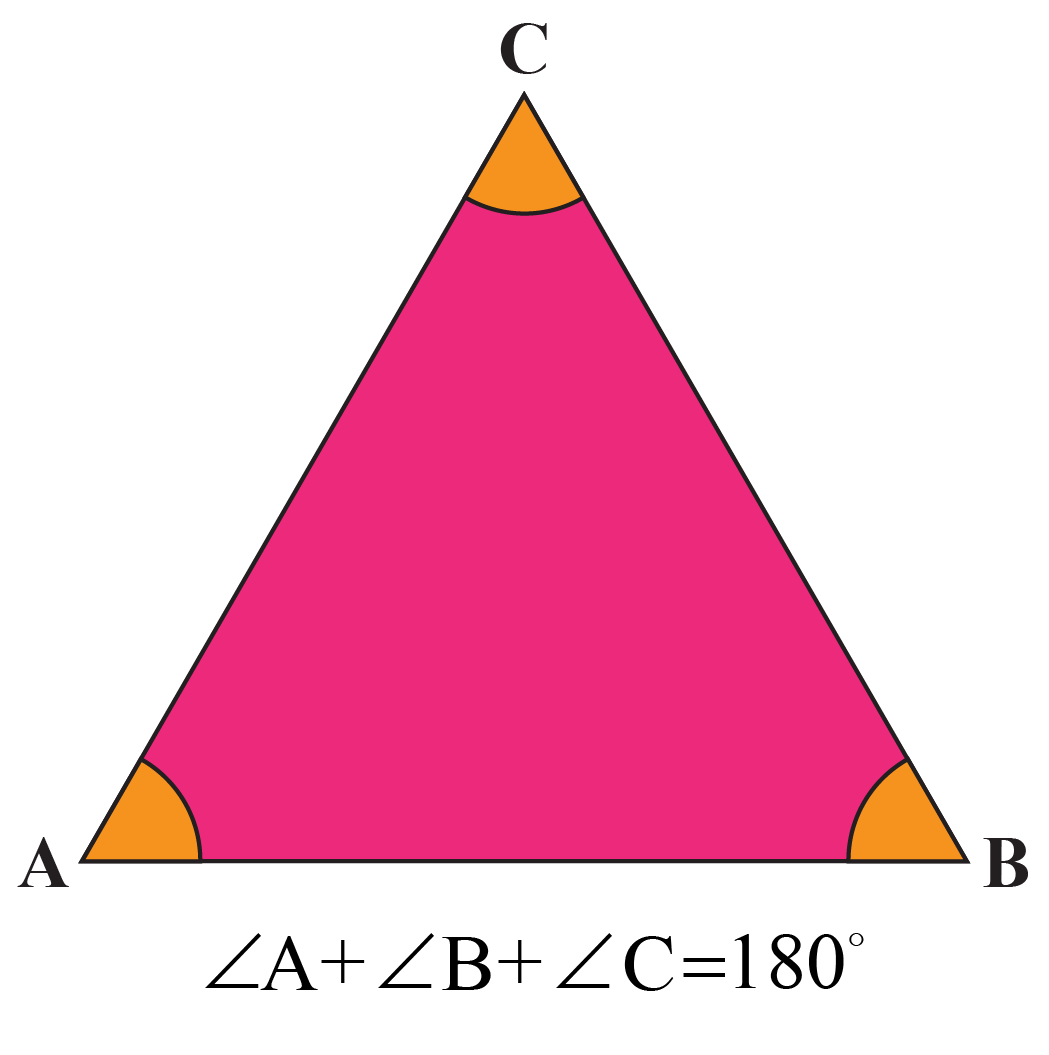Property 3:  Exterior angle of a triangle is equal to the sum of the two opposite interior angles.

A triangle with vertices A, B and C

\begin{align}\angle{1} + \angle{2} = \angle{3}\end{align}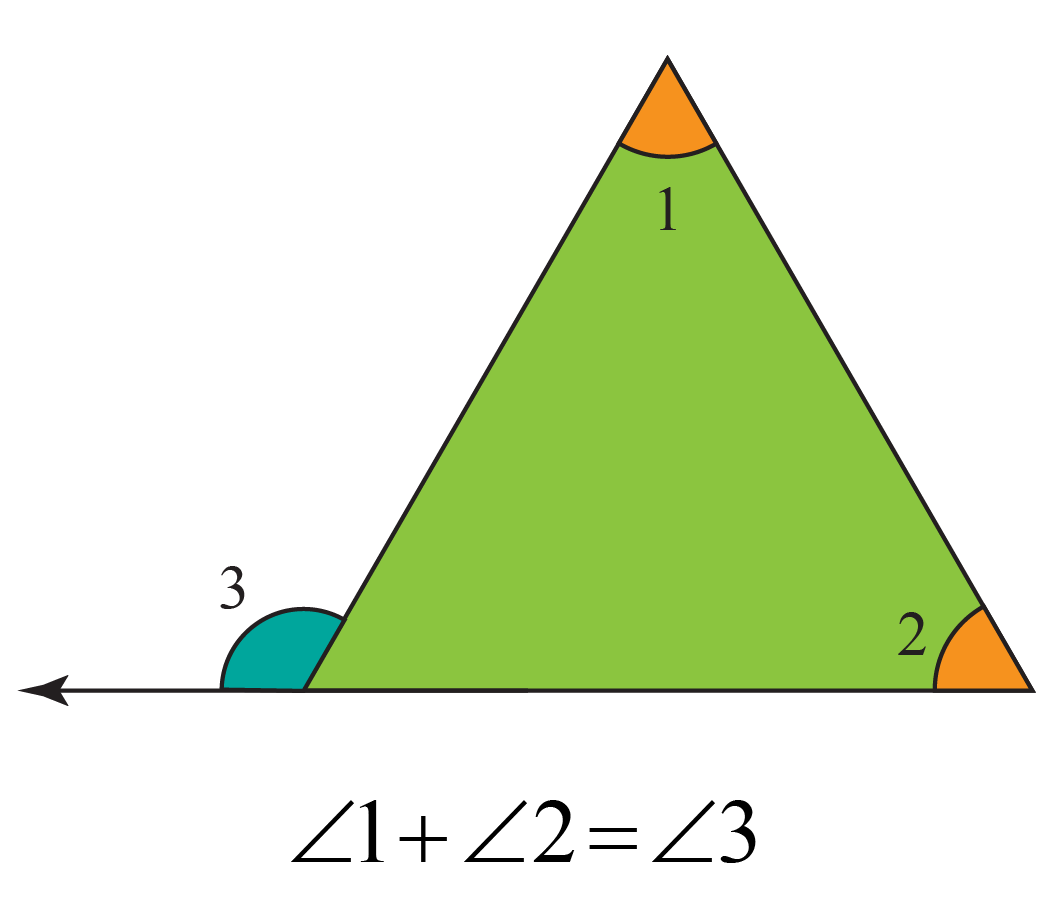where,

\begin{align}\angle{1},\angle{2}\end{align} are interior angles

and

\begin{align}\angle{3}\end{align} is exterior angle.

Property 4: For a right angled triangle, the sum of the square of the hypotenuse is equal to the sum of the squares of the opposite and adjacent sides. This is called as Pythogoras theorem.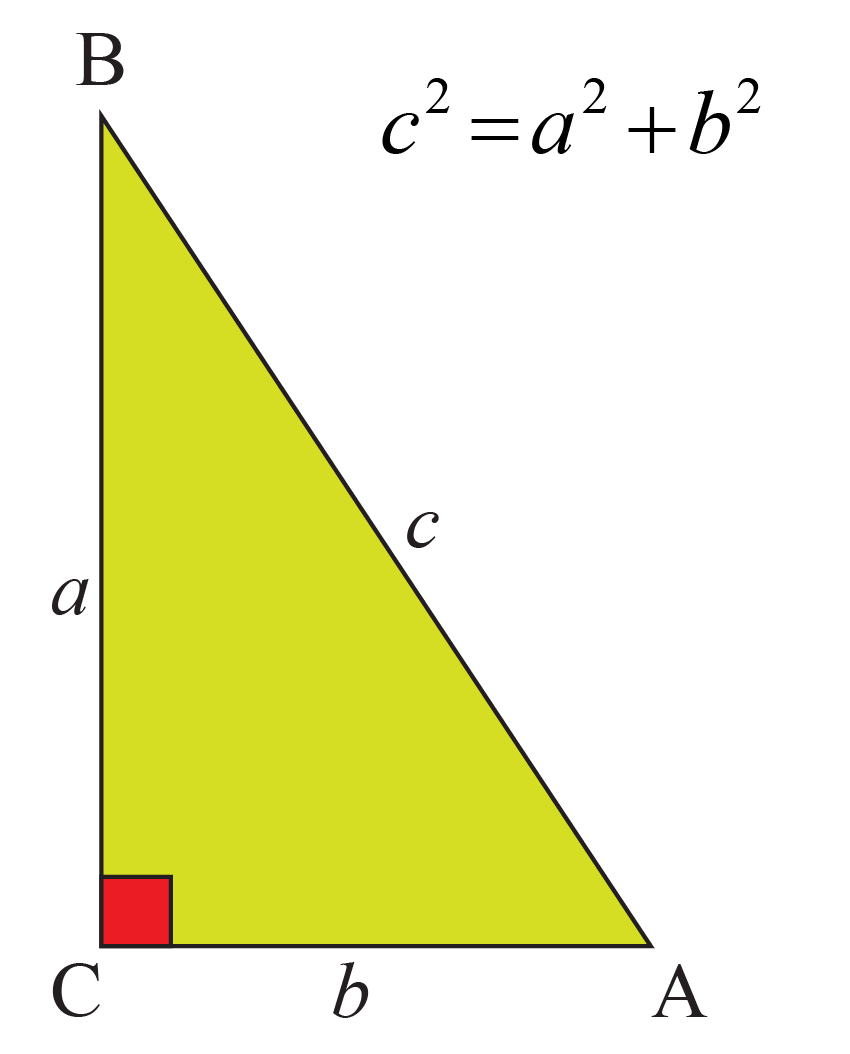A triangle with sides a,b and c has

\begin{align}a^2 + b^2 = c^2\end{align}

### Criteria For Constructing Triangles

Now let us learn the criteria for constructing triangles. There are four important criteria for constructing triangles. They are,

• SSS
• ASA
• SAS
• RHS

### SSS

A triangle that is drawn with the lengths of all the three sides known is a SSS triangle.

### ASA

A triangle drawn with two known angles and one known side is an ASA triangle.

### SAS

A triangle drawn with two known sides and one known angle is a SAS triangle.

### RHS

A triangle drawn with the hypotenuse side (longest side) and another side (can be the opposite side of the adjacent side) is an RHS triangle.

Having learned the properties and criteria now let us construct a triangle ABC with the following measurements.

We will need the following geometrical instruments.

• Ruler
• Compass

AB= 5 units BC = 7 units and AC = 6 units

Step 1:

Draw a line segment BC which measures 7units.

Step 2:

With the point B as center and taking a measure of 5 units in the compass draw an arc.

Step 3:

With the point C as center and taking a measure of 6 units in the compass draw an arc to intersect the arc drawn in step 2.

Step 4:

Now join the intersecting points to B and C as shown below.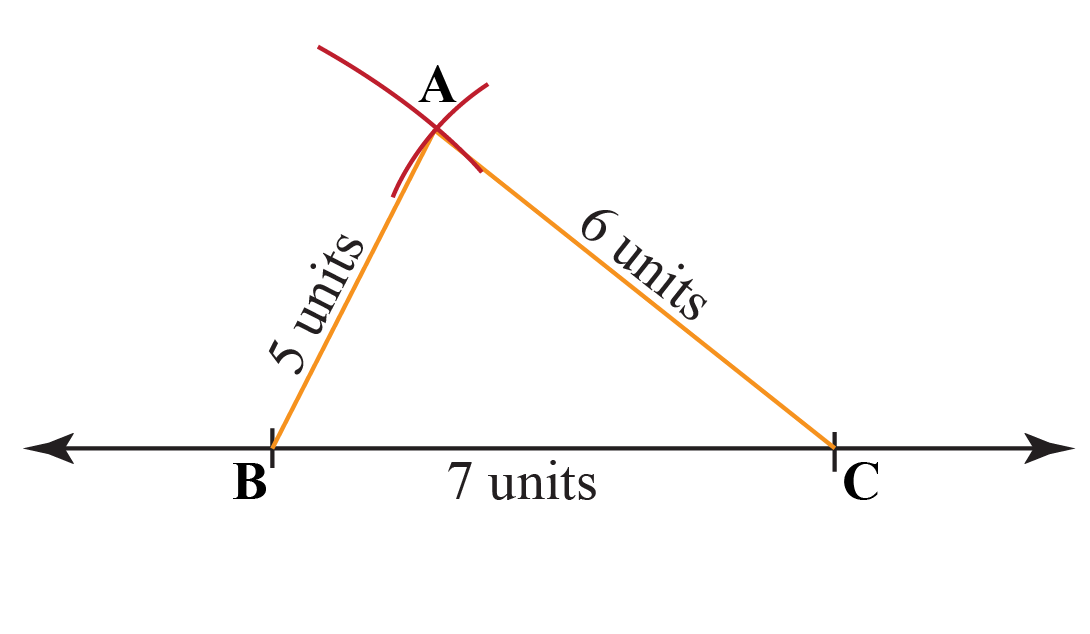The same process suits for equilateral triangle, scalene, and isosceles triangle construction.Think Tank
1. Ron was trying to construct a triangle ABC with BC = 8 units, AB = 3 units and AC = 5 units. But she could not complete it. Can you figure out the reason why he could not do it?2. Are the ratio of angles of a any triangle the same or different? Justify your answer.

 Example 1

Write down the steps in constructing a triangle PRQ with sides PQ = 4.5 units, QR = 6 units and PR = 3.5 units

Solution

Step 1: Draw a line segment QR measuring 6 units.

Step 2: With Q as center, take a measure of 4.5 units in the compass and draw an arc.

Step 3: With R as center, take a measure of 3.5 units in the compass and draw an arc intersecting the previous arc.

Step 4: Connect the lines PQ and PR.

 Thus the triangle PQR is drawn.
 Example 2

Draw an equilateral triangle XYZ  with sides of the triangle equal to 6 units.

Solution

Since we know that an equilateral triangle has all the side lengths to be the same.

Step 1: Draw a line segment YZ = 6 units.

Step 2: With Y as a center and taking a measure of 6 units draw an arc.

Step 3: With Z as center and without changing the measure of length taken in the compass draw another arc to intersect the previous arc.

Step 4: Join the points XY and XZ.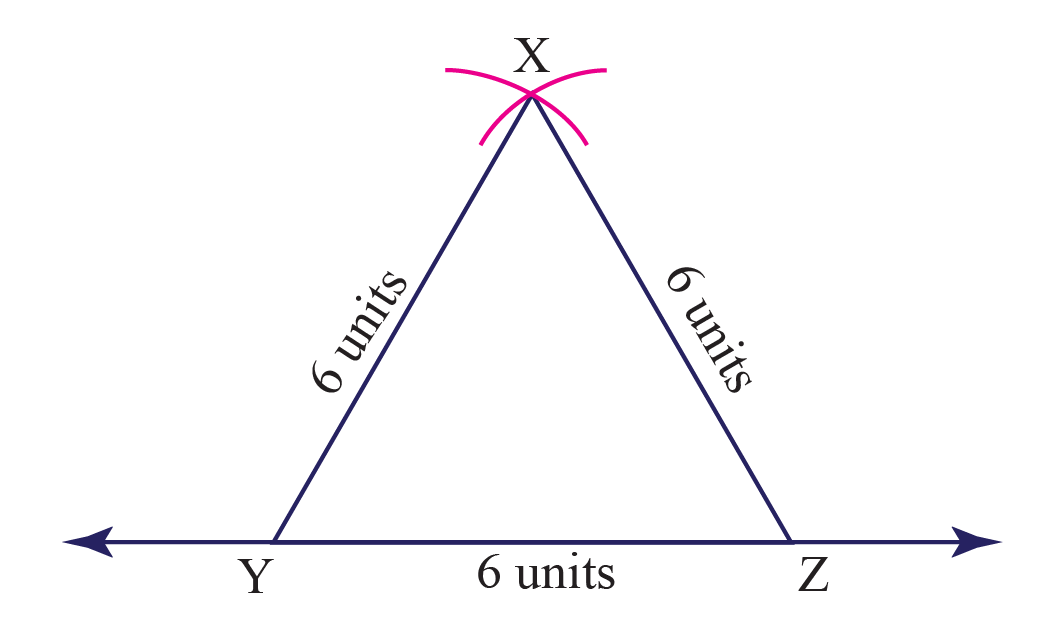Thus the equilateral triangle XYZ is drawn.
 Example 3

Construct a triangle with \begin{align} XY=7\: units \: \angle{X} = 40^0 , \angle{Y} = 105^0\end{align}

Solution

Step 1: Draw a line segment with \begin{align} XY=7\: units \end{align}

Step 2: With X as center, use a protractor to measure \begin{align} \angle{X} = 40^0 \end{align} and draw a ray 'XP'.

Step 3: With Y as center, use a protractor to measure \begin{align} \angle{X} = 105^0 \end{align} and draw a ray 'YQ' such that it intersects with 'XP'. Mark the point of intersection as 'Z'.

Step 4: Join the points ZX and ZY to make the triangle complete.

Please note that, the third angle, which is \begin{align} \angle{Z} \end{align} can be found using the angle sum property of triangles.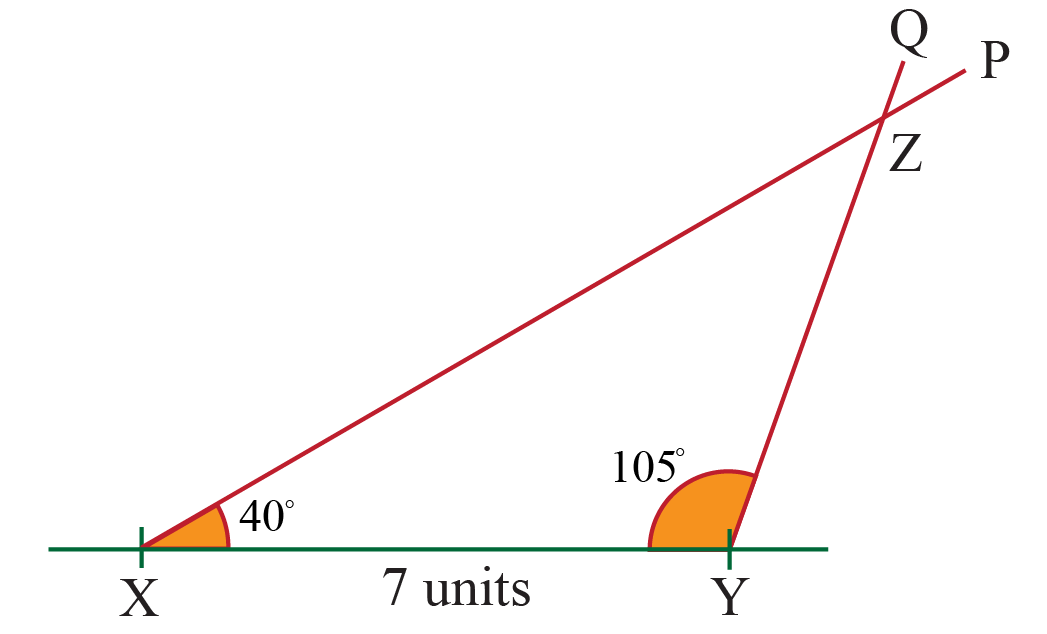Thus the triangle XYZ is constructed

## Interactive Questions

Here are a few activities for you to practice. Select/Type your answer and click the "Check Answer" button to see the result.

Let's Summarize

The lesson targeted the fascinating concept of constructing triangles. The math journey around triangle constructions starts with knowing the basic properties of a triangle. Working out the solved examples and the interactive questions would enrich your knowledge on the subject. Done in a way that not only is relatable and easy to grasp, but also will stay with them forever. Here lies the magic with Cuemath.

At Cuemath, our team of math experts is dedicated to making learning fun for our favorite readers, the students!

Through an interactive and engaging learning-teaching-learning approach, the teachers explore all angles of a topic.

Be it worksheets, online classes, doubt sessions, or any other form of relation, it’s the logical thinking and smart learning approach that we, at Cuemath, believe in.

## FAQs on Triangle Constructions

### 1. How do you construct a triangle with 3 sides?

Draw a line with the length of the longest side. Draw two arcs from the two endpoints of the line drawn such that they intersect each other. Join the intersecting point with the vertices of the longest side.

### 2. How do you draw congruent triangles?

Draw a line with the length of the longest side. Draw two arcs from the two endpoints of the line drawn such that they intersect each other. Join the intersecting point with the vertices of the longest side.

### 3. How do you construct an angle in a triangle?

By placing the protractor on one side of the triangle and marking the required measure of angle

### 4. How do you construct a triangle using a perpendicular bisector and side?

For a line segment draw a perpendicular bisector using a compass and join the point of intersection of the arc with the two vertices of the line segment.

grade 9 | Questions Set 1